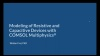# Learning Center Resources

## Modeling Joule Heating in the Time Domain for Resistive and Capacitive Systems

In Part 8 of a 10-part course, Introduction to Modeling Resistive and Capacitive Devices, we discuss modeling transient Joule heating of nonlinear materials under steady-state conditions. Our example model is that of a resistor and capacitor connected to a current source. In starting with the stationary case, we include the Electric Currents and Electrostatics physics interfaces through which we compute the resistance and capacitance of the device, respectively. Upon obtaining the solution, we show you how to evaluate the time constant of the system.

Now we move the current model into the time domain, where we solve for the conduction currents and displacement currents over time. Upon doing so, we show how to compare the results from this time-dependent study to the first, stationary study, which served as the DC case. A withsol operator is used to accomplish this. At this time, we also discuss the relation between the tolerance and the time steps taken by the solver.

Next, we show how to introduce a variation into a simulation that is happening over time. We show two ways through which you can do this:

1. Adding a Boolean expression to our Terminal boundary condition in the Electric Currents interface
2. Using the Events interface

Following this, we add a Frequency-Transient study, and show how to solve the electric currents part of our model in the frequency domain by taking the cycle-averaged losses and passing into the heat transfer part of our model. We then demonstrate how to evaluate quantities such as the voltage, losses, maximum temperature, and variation over time in the device, and create plots for each of these quantities.

Then, we discuss the options for studies that can be added to our model to compute the multiphysics phenomena:

1. Through a coupled approach, using a Frequency-Transient study
2. Through a sequential approach, using a Frequency-Transient, One-Way Electromagnetic Heating study

We also break down how the physics are computed for each study type, discuss use cases for each study type, and create plots to visualize the difference in the solution of the two different studies. Different or additional physics features and settings may be required for using either approach named above to compute the model, which we also cover.